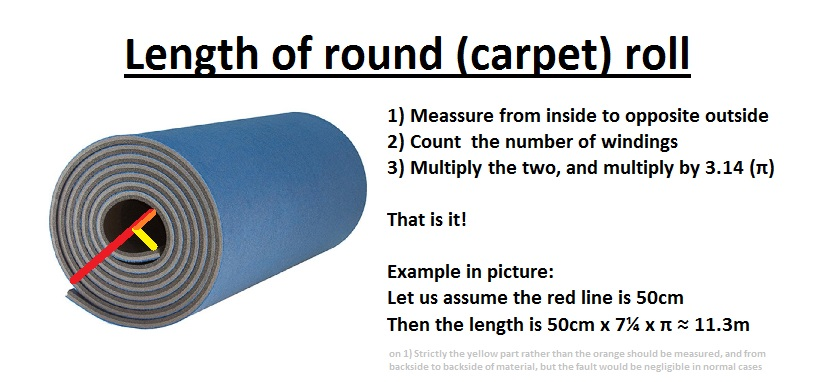# Length of round (carpet) rollFor some reason, surprisingly few know this extremely simple formula, please share it.

I have actually seen in a carpet store an employee unrolling the carpet to measure the length!!

[The formula is simple to prove too… r=kφ, L=∫r dφ=∫kφ dφ=½k(φ2²-φ1²)=½k(φ21)×(φ21)=½(r2+r1) ×n2π=(r2+r1) × nπ , q.e.d.]